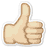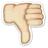Meaning AVERAGE DEMAND
What does AVERAGE DEMAND mean? Here you find 5 meanings of the word AVERAGE DEMAND. You can also add a definition of AVERAGE DEMAND yourself

# 1

11# AVERAGE DEMAND

The amount of energy being consumed in an electric system over an interval in time, as determined by dividing the total number of kilowatt-hours by the number of units of time in the interval.
 Source: greatriverenergy.com

# 2

11# AVERAGE DEMAND

The total demand for water during a period of time divided by the number of days in that time period. This is also called the average daily demand.
 Source: owp.csus.edu

# 3

11# AVERAGE DEMAND

Total amount of the particular unit divide by given periods of time ; fore example in daily term, weekly term or monthly way
 Elizabeth joseph - 5 October 2020

# 4

01# AVERAGE DEMAND

The energy demand for a given location over a period of time. For example, the number of kilowatt-hours used in a 24-hour period, divided by 24 hours, tells the average demand for that location in tha [..]
 Source: solar-estimate.org

# 5

01# AVERAGE DEMAND

The energy demand in a given geographical area over a period of time. For example, the number of kilowatt-hours used in a 24-hour period, divided by 24, tells the average demand for that period. (SOURCE: California Energy Commission, http://www.consumerenergycenter.org)
 Source: aresnorthamerica.com

 Add meaning of AVERAGE DEMAND Word count:
 Name: E-mail: (* optional)

 << Swt MEGAWATTS >>
Dictionary.university is a dictionary written by people like you and me.